# Tubing Length Change due to Buckling

When tubing is freely suspended, it can be buckled by an upward force applied at the bottom of tubing. A section of tubing exposed to compression force will have a chance of being buckled. However, a part which is under tension will not face a buckle issue.

The neutral point is the boundary below which buckling can possibly be occurred and above which buckling will not happen.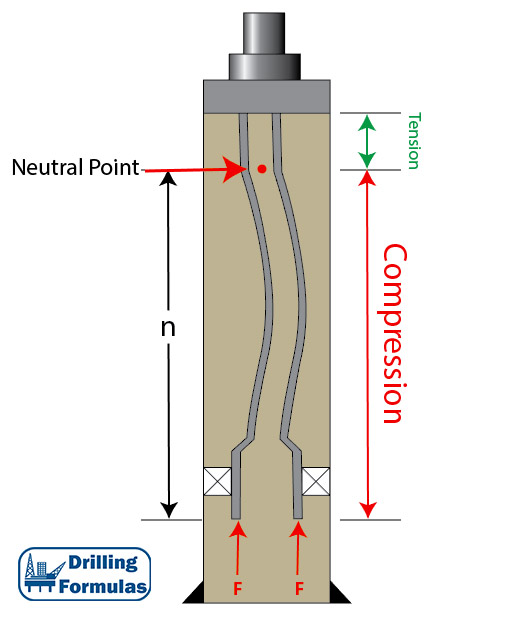Figure 1 – Wellbore Diagram with Tubing Buckling Due to Compression Force

Bulking Calculations

Location of a neutral point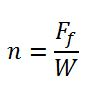If n < L, this indicates a partially buckled and the length change due to buckling is calculated by this formula below;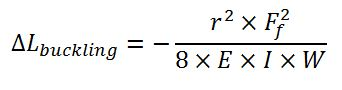If n > L, this indicates a completely buckled and the length change due to buckling is calculated by this formula below;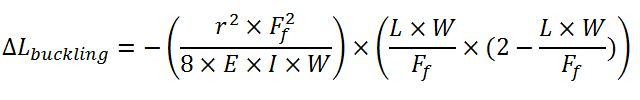W = Ws + Wi – Wo

Wi = ρi × Ai

Wo = ρo × Ao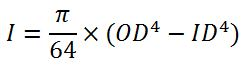Ff = Ap × (Pi – Po)

Where;

Ff = fictitious force or effective buckling force (lb)

Ap = cross section area of packer seal bore (in2)

Pi = Pressure at the packer inside tubing (psi)

Po = Pressure at tubing in the annulus (psi)

I = moment of inertia of tubing cross section (in4)

OD = outside diameter of tubing (in)

ID = inside diameter of tubing (in)

r = radial clearance between tubing OD and casing ID (in)

L = length of tubular (in)

E = Young’ modulus of material (psi)

Ws = weight per unit weight of steel (lb./in)

Wi = weight per unit weight of fluid inside tubing (lb./in)

Wo = weight per unit weight of fluid outside tubing (lb./in)

ρi = density of fluid in the tubing (lb/in3)

ρo = density of fluid in the annulus (lb/in3)

W = weight per unit length of tubular in the presence of fluid (lb/in)

Ff (fictitious force) is a combination of forces from internal pressure, external pressure and piston force. This is not the actual force acting at the bottom of tubing. The Ff (fictitious force) is used to indicate if the pipe is buckled or not. Tubing will be buckled if Ff is positive. If Ff is zero or less than zero, tubing will not be buckled because tubing is in tension (ΔLbuckling = 0).

Example

Packer is set at 10,000 ft.

Tubing and packer are free to move.

The well is vertical.

4.5” Tubing (15.1 lb/ft.)

ID of tubing = 3.826”

Packer seal bore outside diameter = 5.0”

Weight per length = 17.7 lb/ft.

E (Young’s modulus) = 30 × 106

µ (Poisson’s ratio) = 0.3

Production casing size = 7”

Production casing ID = 6.049”

Initial Condition

Fluid in annulus = 10.0 ppg

Fluid in tubing = 10.0 ppg

Tubing pressure = 0 psi

Annulus pressure = 0 psi

Final Condition

Fluid in annulus = 10.0 ppg

Fluid in tubing = 8.0 ppg

Tubing pressure = 1,500 psi

Annulus pressure = 0 psiFigure 2 – Initial and Final Condition

Solution

For bulking calculation, the final condition of the tubing is considered.

For the analysis, Lubinski’s sign conventions are used. Please see at the reference section to get a full paper.

Compression force = ⇑(+)

Tensile force = ⇓(-)

Shorten in Length = (-)

Elongate in length = (+)

Calculate Areas

Ai = Tubing ID area (in2)

Ai= (π÷4) × 3.8262 = 11.497 in2

Ao = Tubing OD area (in2)

Ao= (π÷4) × 4.52 = 15.904 in2

Ap = Packer sealbore area (in2)

Ap= (π÷4) × 52 = 19.635 in2

Calculate Pressure

Pressure at the packer inside tubing (Pi)

Pi = surface pressure + hydrostatic pressure

Pi = 1,500 + (0.052 ×8 ×10,000) = 5,660 psi

Pressure at the packer in the annulus (P0)

P0 = surface pressure + hydrostatic pressure

P0 = 0 + (0.052 ×10 ×10,000) = 5,200 psi

Calculate Ff (Fictitious Force)

Ff = Ap × (Pi – Po)

Ff = 19.635 × (5,660 – 5,200)

Ff= 9,032 lb

Calculate W

W = Ws + Wi – Wo

Wi = ρi × Ai

Wo = ρo × Ao

ρi = 8 ppg = 8×4.329 ×10-3 = 0.034632 lb/in3

ρo = 10 ppg = 10×4.329 ×10-3 = 0.04329 lb/in3

Ws = 15.5÷12 = 1.292 lb/in

Wi = 0.034632 ×11.497 = 0.3982 lb/in

Wo = 0.04329 × 15.904 = 0.6885 lb/in

W = 1.292 + 0.3982 – 0.6885= 1.0013 lb/in

Calculate nn = 9,093÷1.0013= 9,020.1 inch

Compare n and L

n = 9,020.1 inch

L = 10,000 × 12 = 120,000 inch

n<L

If n < L, this indicates a partially buckled and the length change due to buckling is calculated by this formula below;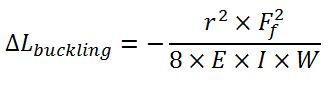r = (6.049 – 4.5) ÷ 2 = 0.7745  inch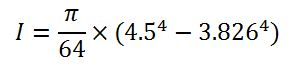I = 9.61 in4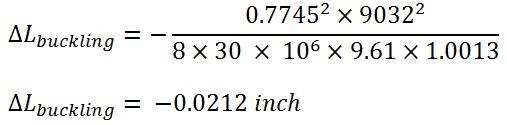Conclusion

The tubing is shorten 0.0212 inch.

References

Jonathan Bellarby, 2009. Well Completion Design, Volume 56 (Developments in Petroleum Science). 1 Edition. Elsevier Science.

Wan Renpu, 2011. Advanced Well Completion Engineering, Third Edition. 3 Edition. Gulf Professional Publishing.

Ted G. Byrom, 2014. Casing and Liners for Drilling and Completion, Second Edition: Design and Application (Gulf Drilling Guides). 2 Edition. Gulf Professional Publishing.

Lubinski, A., & Althouse, W. S. (1962, June 1). Helical Buckling of Tubing Sealed in Packers. Society of Petroleum Engineers. doi:10.2118/178-PA

Share the joy
Tagged , , , , , . Bookmark the permalink.